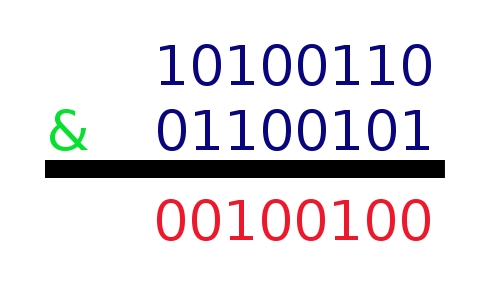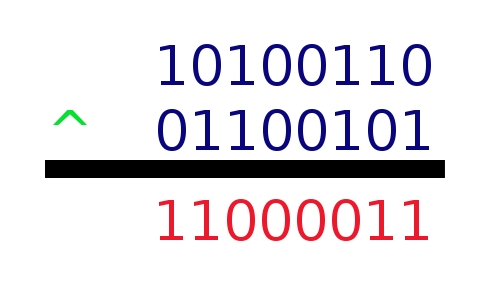# Article 36 - Programming Bits

Bits can be an intimitating programming concept but once you grasp the fundamentals, it is a very simple concept. There are a few key things to remember, bit vector, bit shifting, and bit manipulation.

## Bit Vector

A bit vector is not a difficult concept. In fact, if you are familiar with low level computer concepts and architectures, it should be easy for you to understand. A bit vector is simply an array of bits. It is that simple. Except this array of bits is normally represented as an unsigned integer to reduce memory consumption.

We will use the case of an 8-bit machine, thus the unsigned integer is 1 byte, which is 8 bits. Thus, a bit vector represented by an int is an array of 8 bits. Each bit is represented by a particular combination of values of the integer.

For example, the integer 1 is the bit vector 00000001.

The integer 2 is the bit vector 000000010.

The integer 3 is the bit vector 000000011.

If you noticed, the bit vector is simply the binary representation of the unsigned integer.

## Bit Shifting

Bit shifting is another very simple concept. The idea is to shift the array of bits. For example, in Java, to bit shift left by 1 slot, we do this "00000001 << 1". This produces 00000010.

A key way to check is that each shift to the left doubles the value, while each shift to the right halves the value.

Simply take the number on the right side, and shift the ways in the array that many times in the direction of the arrows.

## Bit Manipulation

There are many methods to manipulate a bit array. It is often used for conditional checks, called bit masking.

Suppose we have the two bit arrays, 00101100 and 10101010. Applying the mask & (and), we get 00101000. We return a 1 in the case where both bits are 1 in that slot, otherwise we return a 0. It is just the simple "and" defintion.

The other bit masks includes XOR (^), and OR (|). XOR, exclusive or, returns a 1 if either bit is 1, but not both. The OR is just the normal defintion, return a 1 if either of the bits is 1.Applying the AND operation to two bit vectors.Applying the OR operation to two bit vectors.Applying the XOR operation to two bit vectors.

## Posted by anonymous - ciprofloxacin hcl at Friday, March 15, 2013 10:49 AM

Im thankful for the article.Much thanks again.

## Posted by anonymous - generic viagra no prescription at Friday, March 15, 2013 12:28 PM

I value the blog article.Really looking forward to read more. Cool.

## Posted by anonymous - buy generic cialis at Friday, March 15, 2013 2:08 PM

Im thankful for the blog article.Really looking forward to read more. Great.

## Posted by anonymous - 20mg levitra at Friday, March 15, 2013 3:48 PM

Thank you for your blog article.Really thank you! Awesome.

## Posted by anonymous - autolaina at Monday, March 25, 2013 7:13 AM

Thanks for the laughs while wandering down memory lane! Geez, now I feel old too. autolaina http://www.wandmaaponiock.com